#### Generating random directions

Some sampling-based algorithms require choosing motion directions at random.5.4 From a configuration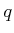, the possible directions of motion can be imagined as being distributed around a sphere. In an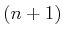-dimensional C-space, this corresponds to sampling on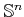. For example, choosing a direction in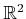amounts to picking an element of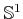; this can be parameterized as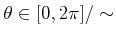. If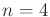, then the previously mentioned trick for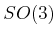should be used. If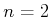or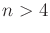, then samples can be generated using a slightly more expensive method that exploits spherical symmetries of the multidimensional Gaussian density function . The method is explained for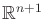; boundaries and identifications must be taken into account for other spaces. For each of the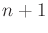coordinates, generate a samplefrom a zero-mean Gaussian distribution with the same variance for each coordinate. Following from the Central Limit Theorem,can be approximately obtained by generatingsamples at random over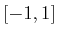and adding them (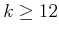is usually sufficient in practice). The vector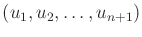gives a random direction inbecause eachwas obtained independently, and the level sets of the resulting probability density function are spheres. We did not use uniform random samples for eachbecause this would bias the directions toward the corners of a cube; instead, the Gaussian yields spherical symmetry. The final step is to normalize the vector by taking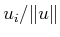for each coordinate.

Steven M LaValle 2020-08-14